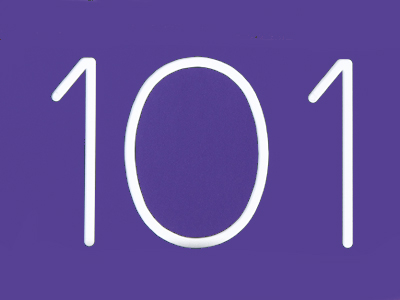The best way to multiply 93 by 101 is to multiply it by 100 and then add 93.

# Multiplication and Division 4

This Math quiz is called 'Multiplication and Division 4' and it has been written by teachers to help you if you are studying the subject at elementary school. Playing educational quizzes is an enjoyable way to learn if you are in the 3rd, 4th or 5th grade - aged 8 to 11.

It costs only \$12.50 per month to play this quiz and over 3,500 others that help you with your school work. You can subscribe on the page at Join Us

Multiplication and division (specifically) use remainders. Remainders are parts of a number that are left over. As an example, I have 4 cats and 10 cat treats. I can only give each cat 2 treats each, which means I will have 2 treats left over. The 2 left can't be divided by 4 as it is below 4, therefore the 2 is a 'remainder'. Remainders can be converted into fractions and decimals like so:
10 ÷ 4 = 2 r2 or 2.25 or 214

1.
5.87 x 100 = ?
587
5870
0.587
0.0587
To multiply a number by 100 move its decimal point 2 places to the right
2.
Which strategy would be best to multiply 53 by 25?
Multiply by 100 then double
Multiply by 4 then 100
Multiply by 100 then divide by 4
Divide by 4 then double
Just as 0.25 is 14 of 1, 25 is 14 of 100
3.
2.43 x 10 = ?
2.430
24.3
243
0.243
To multiply a number by 10 move its decimal point 1 place to the right
4.
Which strategy would be best to multiply 93 by 101?
Multiply by 10 then add 93
Multiply by 100 then add 93
Multiply by 100 then subtract 93
Multiply by 10 then subtract 93
You can use this strategy for other numbers over 100, for example to multiply a number by 107 multiply by 100 and also by 7 then add the 2 results together
5.
(6 + 5) x (8 - 3) + 6 =
(6 + 5 x 8) - (3 + 6) =
6 + 5 x (8 - 3) + 6 =
(6 + 5) x 8 - 3 + 6 =
Work out the calculations in brackets first
6.
On a calculator the answer to a division question is 5.25. What fraction is the remainder?
Half
Quarter
Third
Eighth
0.25 is the decimal equivalent of 14
7.
What is the best method of multiplying 81 by 99?
Multiply by 100 then subtract 81
Multiply by 10 then add 81
Multiply by 100 then add 81
Multiply by 10 then subtract 81
Multiplying by 100 is much easier than multiplying by 99
8.
Which of these methods would result in finding 112?
Half one third twice
Half one quarter twice
Double one quarter twice
Double one sixth
e.g. 112 of 66. One third is 22, half of 22 is 11, half of 11 is 5.5
9.
How could we find an eighth of a number?
Multiply it by 4
Multiply it by 8
Divide it by 8
Divide it by 4
An eighth of a number is the same as saying divide it by 8
10.
What is the best method of multiplying 72 by 15?
Multiply by 10 then halve
Multiply by 10, halve the result, then add the two parts together
Multiply by 10, divide by 5, then add the two numbers
Divide by 10 then double
72 x 10 = 720; half of 720 = 360, so 720 + 360 = 1,080
Author:  Amanda Swift